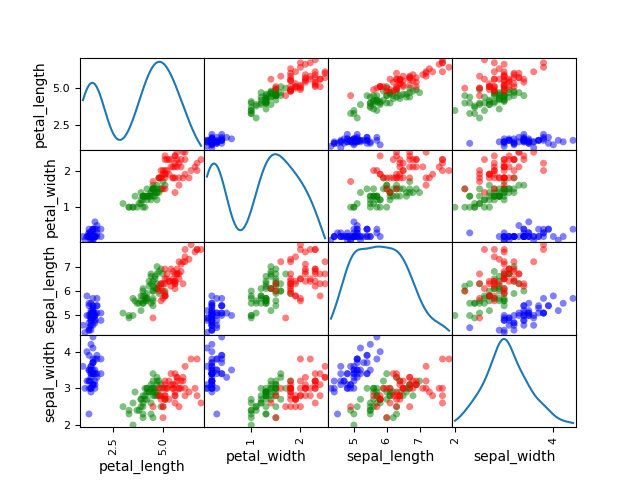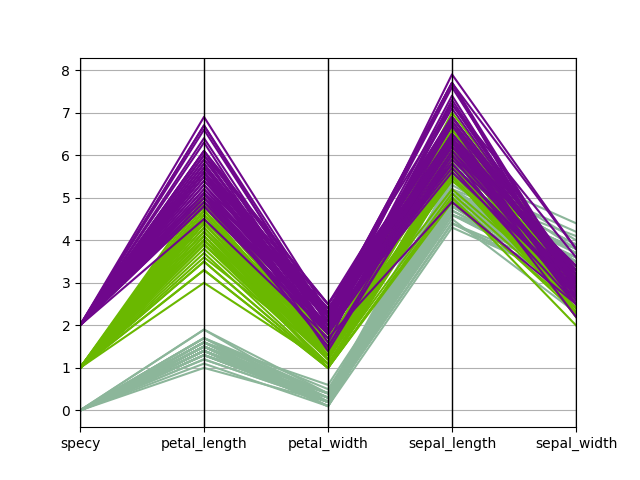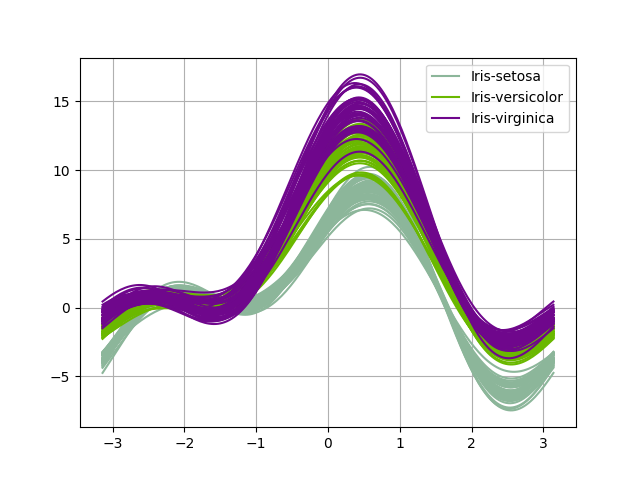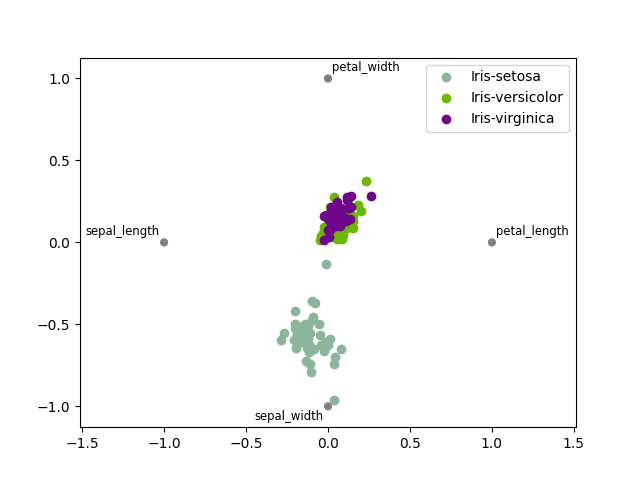# Iris dataset¶

## Presentation¶

This is one of the best known dataset to be found in the machine learning literature.

It was introduced by the statistician Ronald Fisher in his 1936 paper “The use of multiple measurements in taxonomic problems”, Annals of Eugenics. 7 (2): 179–188.

It contains 150 instances of iris plants:

• 50 Iris Setosa,

• 50 Iris Versicolour,

• 50 Iris Virginica.

Each instance is characterized by:

• its sepal length in cm,

• its sepal width in cm,

• its petal length in cm,

• its petal width in cm.

This dataset can be used for either clustering purposes or classification ones.

from __future__ import annotations

from gemseo.api import configure_logger
from gemseo.post.dataset.andrews_curves import AndrewsCurves
from gemseo.post.dataset.parallel_coordinates import ParallelCoordinates
from gemseo.post.dataset.scatter_plot_matrix import ScatterMatrix
from numpy.random import choice

configure_logger()

<RootLogger root (INFO)>


We can easily load this dataset by means of the load_dataset() function of the API:

iris = load_dataset("IrisDataset")


and get some information about it

print(iris)

Iris
Number of samples: 150
Number of variables: 5
Variables names and sizes by group:
labels: specy (1)
parameters: petal_length (1), petal_width (1), sepal_length (1), sepal_width (1)
Number of dimensions (total = 5) by group:
labels: 1
parameters: 4


## Manipulate the dataset¶

We randomly select 10 samples to display.

shown_samples = choice(iris.length, size=10, replace=False)


If the pandas library is installed, we can export the iris dataset to a dataframe and print(it.

dataframe = iris.export_to_dataframe()
print(dataframe)

    labels   parameters
specy petal_length petal_width sepal_length sepal_width
0            0           0            0           0
0      0.0          1.4         0.2          5.1         3.5
1      0.0          1.4         0.2          4.9         3.0
2      0.0          1.3         0.2          4.7         3.2
3      0.0          1.5         0.2          4.6         3.1
4      0.0          1.4         0.2          5.0         3.6
..     ...          ...         ...          ...         ...
145    2.0          5.2         2.3          6.7         3.0
146    2.0          5.0         1.9          6.3         2.5
147    2.0          5.2         2.0          6.5         3.0
148    2.0          5.4         2.3          6.2         3.4
149    2.0          5.1         1.8          5.9         3.0

[150 rows x 5 columns]


We can also easily access the 10 samples previously selected, either globally

data = iris.get_all_data(False)
print(data[shown_samples, :])

[[0.  5.  3.2 1.2 0.2]
[2.  6.8 3.2 5.9 2.3]
[1.  6.  2.2 4.  1. ]
[2.  6.  3.  4.8 1.8]
[1.  6.  3.4 4.5 1.6]
[0.  4.9 3.1 1.5 0.2]
[2.  7.3 2.9 6.3 1.8]
[2.  6.2 3.4 5.4 2.3]
[0.  5.4 3.4 1.7 0.2]
[2.  5.8 2.7 5.1 1.9]]


or only the parameters:

parameters = iris.get_data_by_group("parameters")
print(parameters[shown_samples, :])

[[5.  3.2 1.2 0.2]
[6.8 3.2 5.9 2.3]
[6.  2.2 4.  1. ]
[6.  3.  4.8 1.8]
[6.  3.4 4.5 1.6]
[4.9 3.1 1.5 0.2]
[7.3 2.9 6.3 1.8]
[6.2 3.4 5.4 2.3]
[5.4 3.4 1.7 0.2]
[5.8 2.7 5.1 1.9]]


or only the labels:

labels = iris.get_data_by_group("labels")
print(labels[shown_samples, :])

[[0.]
[2.]
[1.]
[2.]
[1.]
[0.]
[2.]
[2.]
[0.]
[2.]]


## Plot the dataset¶

Lastly, we can plot the dataset in various ways. We will note that the samples are colored according to their labels.

### Plot scatter matrix¶

We can use the ScatterMatrix plot where each non-diagonal block represents the samples according to the x- and y- coordinates names while the diagonal ones approximate the probability distributions of the variables, using either an histogram or a kernel-density estimator.

ScatterMatrix(iris, classifier="specy", kde=True).execute(save=False, show=True)/home/docs/checkouts/readthedocs.org/user_builds/gemseo/envs/4.3.0.post0/lib/python3.9/site-packages/gemseo/post/dataset/scatter_plot_matrix.py:135: UserWarning: To output multiple subplots, the figure containing the passed axes is being cleared.
sub_axes = scatter_matrix(

[<Figure size 640x480 with 16 Axes>]


### Plot parallel coordinates¶

We can use the ParallelCoordinates plot, a.k.a. cowebplot, where each samples is represented by a continuous straight line in pieces whose nodes are indexed by the variables names and measure the variables values.

ParallelCoordinates(iris, "specy").execute(save=False, show=True)[<Figure size 640x480 with 1 Axes>]


### Plot Andrews curves¶

We can use the AndrewsCurves plot which can be viewed as a smooth version of the parallel coordinates. Each sample is represented by a curve and if there is structure in data, it may be visible in the plot.

AndrewsCurves(iris, "specy").execute(save=False, show=True)[<Figure size 640x480 with 1 Axes>]


We can use the Radar plot
Radar(iris, "specy").execute(save=False, show=True)[<Figure size 640x480 with 1 Axes>]